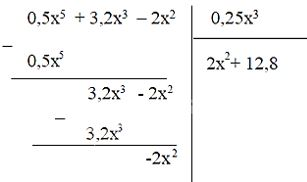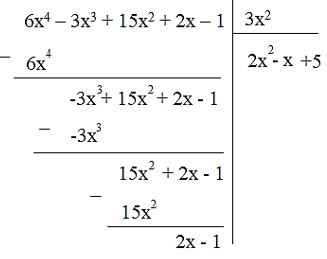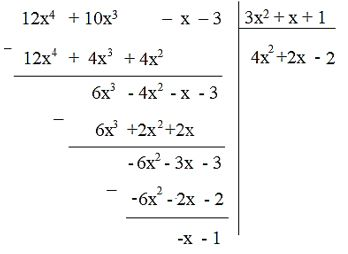## Detailed instructions for solving Problem 7.33

Solution method

To divide a polynomial by a monomial, we divide each term of the polynomial by the monomial and then sum the results.

Detailed explanation

a) (0.5x5 + 3.2x3 – 2x2 ) : 0.25x2

= 0.5x5 : 0.25x2 + 3.2x3 : 0.25x2 + (2x2 : 0.25x2)

= (0.5:0.25).(x5 : x2) + (3.2 : 0.25). (x3 : x2 ) + (2 : 0.25). (x2 : x2)

= 2x3 + 12.8x + 4

b) (0.5x5 + 3.2x3 – 2x2 ) : 0.25x3–>

— *****

### Solution 7.34 page 43 Math textbook 7 Connecting knowledge volume 2 – KNTT

In each of the following cases, find the quotient Q(x) and the remainder R(x) in dividing F(x) by G(x) and express F(x) as:

F(x) = G(x) . Q(x) + R(x)

a) F(x) = 6x4 – 3x3 + 15x2 + 2x – 1 ; G(x) = 3x2

b) F(x) = 12x4 + 10x3 – x – 3 ; G(x) = 3x2 + x + 1

## Detailed instructions for solving problem 7.34

Solution method

+) To divide polynomial A by polynomial B, we do the following:

Step 1: Set the divisibility similar to dividing two natural numbers. Divide the highest-order term of A by the highest-order term of B.

Step 2: Take A minus the product of B with the new quotient obtained in step 1

Step 3: Divide the highest order term of the first remainder by the highest term of B

Step 4: Subtract the product B from the first remainder with the quotient obtained in step 3

Step 5: Do the same as above

When the last remainder is of degree less than the degree of B, the division ends.

+) Write A = B. Q + R

Detailed explanation

a)quotient Q(x) = 2x2 – x + 5

Residual R(x) = 2x – 1

We have: F(x) = 3x2 . (2x .)2 – x + 5) + 2x – 1

b)Quotient Q(x) = 4x2 + 2x – 2

Residual R(x) = -x – 1

We have: F(x) = (3x2 + x + 1) . (4x .)2 + 2x – 2) – x – 1

–>

— *****

### Solve problem 7.35 page 43 Math textbook 7 Connecting knowledge volume 2 – KNTT

Tam is confused when he wants to find the quotient and remainder in the division of the polynomial 21x – 4 by 3x2 . Can I help you Tam?

## Detailed instructions for solving problem 7.35

Solution method

When the division polynomial has degree less than the degree of the divisor, the quotient is 0, the remainder is the divisor

Detailed explanation

Divide polynomial 21x – 4 by 3x2 quotient is 0, remainder 21x – 4

–>

— *****